CAT  >  Test: Venn Diagrams- 5

# Test: Venn Diagrams- 5 - CAT

Test Description

## 30 Questions MCQ Test Logical Reasoning (LR) and Data Interpretation (DI) - Test: Venn Diagrams- 5

Test: Venn Diagrams- 5 for CAT 2023 is part of Logical Reasoning (LR) and Data Interpretation (DI) preparation. The Test: Venn Diagrams- 5 questions and answers have been prepared according to the CAT exam syllabus.The Test: Venn Diagrams- 5 MCQs are made for CAT 2023 Exam. Find important definitions, questions, notes, meanings, examples, exercises, MCQs and online tests for Test: Venn Diagrams- 5 below.
Solutions of Test: Venn Diagrams- 5 questions in English are available as part of our Logical Reasoning (LR) and Data Interpretation (DI) for CAT & Test: Venn Diagrams- 5 solutions in Hindi for Logical Reasoning (LR) and Data Interpretation (DI) course. Download more important topics, notes, lectures and mock test series for CAT Exam by signing up for free. Attempt Test: Venn Diagrams- 5 | 30 questions in 30 minutes | Mock test for CAT preparation | Free important questions MCQ to study Logical Reasoning (LR) and Data Interpretation (DI) for CAT Exam | Download free PDF with solutions
 1 Crore+ students have signed up on EduRev. Have you?
Test: Venn Diagrams- 5 - Question 1

### In the following diagram the boys who are athletic and are disciplined are indicated by which number?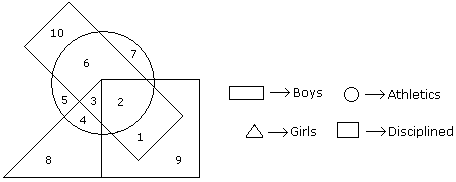Detailed Solution for Test: Venn Diagrams- 5 - Question 1

The required number is 2 because its common in the three shapes.

Test: Venn Diagrams- 5 - Question 2

### In the following figure triangle represents 'girls', square players and circle-coach. Which part of the diagram represents the girls who are player but not coach?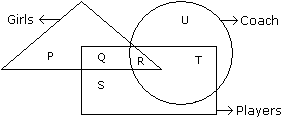Detailed Solution for Test: Venn Diagrams- 5 - Question 2

Q part of the figure represents those girls who are players but not coach.

Test: Venn Diagrams- 5 - Question 3

### In an organization of pollution control board, engineers are represented by a circle, legal experts by a square and environmentalist by a triangle. Who is most represented in the board as shown in the following figure ?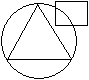Detailed Solution for Test: Venn Diagrams- 5 - Question 3

Triangle represents both environmentalist who are also engineers.So,most represented in the board is environmentalists with engineering background.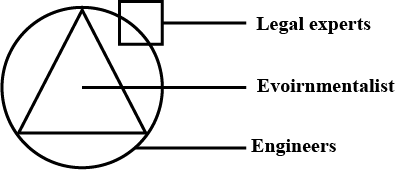Test: Venn Diagrams- 5 - Question 4

The diagram given below represents those students who play Cricket, Football and Kabaddi.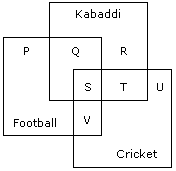Study the diagram and identify the students who play all the three games.

Detailed Solution for Test: Venn Diagrams- 5 - Question 4

S indicates those students who play all three games.

Test: Venn Diagrams- 5 - Question 5

In the figure given below, square represents doctors, triangle represents ladies and circle represents surgeon. By which letter the ladies who doctor and surgeon both are represented ?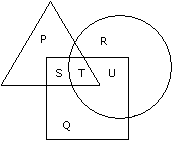Detailed Solution for Test: Venn Diagrams- 5 - Question 5

Correct option is B)

No answer description available for this question.

Test: Venn Diagrams- 5 - Question 6

Study the diagram and identify the people who can speak only one language.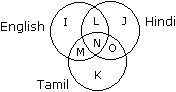Detailed Solution for Test: Venn Diagrams- 5 - Question 6

The regions represented by the letters K, J and I denote such people who can speak only one language.

Test: Venn Diagrams- 5 - Question 7

In the given figure if Triangle represents healthy people, Square represents old persons and Circle represents men then What is the number of those men who are healthy but not old ?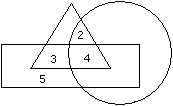Detailed Solution for Test: Venn Diagrams- 5 - Question 7

Correct option is D)

No answer description available for this question.

Test: Venn Diagrams- 5 - Question 8

Directions to Solve

In the following figure small square represents the persons who know English, triangle to those who know Marathi, big square to those who know Telugu and circle to those who know Hindi. In the different regions of the figures from 1 to 12 are given.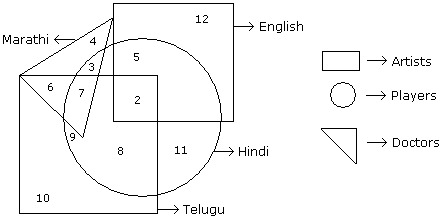Question -

How many persons can speak English and Hindi both the languages only ?

Detailed Solution for Test: Venn Diagrams- 5 - Question 8

Number of persons who can speak English and Hindi both only is 5.

Test: Venn Diagrams- 5 - Question 9

Directions to Solve

In the following figure small square represents the persons who know English, triangle to those who know Marathi, big square to those who know Telugu and circle to those who know Hindi. In the different regions of the figures from 1 to 12 are given.Question -

How many persons can speak Marathi and Telugu both ?

Detailed Solution for Test: Venn Diagrams- 5 - Question 9

Explanation:
6 + 7 = 13.

Test: Venn Diagrams- 5 - Question 10

Directions to Solve

In the following figure small square represents the persons who know English, triangle to those who know Marathi, big square to those who know Telugu and circle to those who know Hindi. In the different regions of the figures from 1 to 12 are given.Question -

How many persons can speak English, Hindi and Telugu ?

Detailed Solution for Test: Venn Diagrams- 5 - Question 10

Number of persons who can speak English, Hindi and Telugu is 2.

Test: Venn Diagrams- 5 - Question 11

Directions to Solve

In the following figure small square represents the persons who know English, triangle to those who know Marathi, big square to those who know Telugu and circle to those who know Hindi. In the different regions of the figures from 1 to 12 are given.Question -

How many persons can speak only English ?

Detailed Solution for Test: Venn Diagrams- 5 - Question 11

Number of persons were can speak English is 12.

Test: Venn Diagrams- 5 - Question 12

Directions to Solve

In the following figure small square represents the persons who know English, triangle to those who know Marathi, big square to those who know Telugu and circle to those who know Hindi. In the different regions of the figures from 1 to 12 are given.Question -

How many persons can speak all the languages ?

Detailed Solution for Test: Venn Diagrams- 5 - Question 12

There is no such person who can speak all the languages.

Test: Venn Diagrams- 5 - Question 13

Directions to Solve

Study the diagram given below and answer each of the following questions.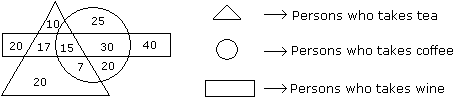Question -

How many persons who take tea and wine but not coffee ?

Detailed Solution for Test: Venn Diagrams- 5 - Question 13

17 persons take tea and wine but not coffee.

Test: Venn Diagrams- 5 - Question 14

Directions to Solve

Study the diagram given below and answer each of the following questions.Question -

How many persons are there who take both tea and coffee but not wine ?

Detailed Solution for Test: Venn Diagrams- 5 - Question 14

Number of persons was taken both tea and coffee but not wine is 7.

Test: Venn Diagrams- 5 - Question 15

Directions to Solve

Study the diagram given below and answer each of the following questions.Question -

How many persons are there who takes only coffee ?

Detailed Solution for Test: Venn Diagrams- 5 - Question 15

25 + 20 = 45

Test: Venn Diagrams- 5 - Question 16

Directions to Solve

Study the diagram given below and answer each of the following questions.Question -

How many persons take wine ?

Detailed Solution for Test: Venn Diagrams- 5 - Question 16

122 persons take wine.

Test: Venn Diagrams- 5 - Question 17

Directions to Solve

Study the diagram given below and answer each of the following questions.Question -

How many persons takes all the three ?

Detailed Solution for Test: Venn Diagrams- 5 - Question 17

15 persons take all the three.

Test: Venn Diagrams- 5 - Question 18

Directions to Solve

Study the following figure and answer the questions given below.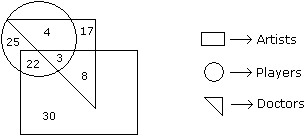Question -

How many doctors are neither artists nor players ?

Detailed Solution for Test: Venn Diagrams- 5 - Question 18

The number of doctors who are neither artists nor players is 17.

Test: Venn Diagrams- 5 - Question 19

Directions to Solve

Study the following figure and answer the questions given below.Question -

How many doctors are both players and artists ?

Detailed Solution for Test: Venn Diagrams- 5 - Question 19

The number of doctors who are both players and artists is 3.

Test: Venn Diagrams- 5 - Question 20

Directions to Solve

Study the following figure and answer the questions given below.Question -

How many artists are players ?

Detailed Solution for Test: Venn Diagrams- 5 - Question 20

The number of artists who are players is 22 + 3 = 25.

Test: Venn Diagrams- 5 - Question 21

Directions to Solve

Study the following figure and answer the questions given below.Question -

How many players are neither artists nor doctors ?

Detailed Solution for Test: Venn Diagrams- 5 - Question 21

The number of players who are neither artists nor doctors is 25.

Test: Venn Diagrams- 5 - Question 22

Directions to Solve

Study the following figure and answer the questions given below.Question -

How many artists are neither players nor doctors ?

Detailed Solution for Test: Venn Diagrams- 5 - Question 22

The number of artists who are neither players nor doctors is 30.

Test: Venn Diagrams- 5 - Question 23

Directions to Solve

In the following diagram rectangle represents men, Triangle represents educated, Circle represents urban and square represents government employees.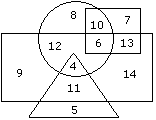Question -

Which one of the following represents the educated men but not urban ?

Detailed Solution for Test: Venn Diagrams- 5 - Question 23

The logic follows here is:

Educated men who are not urban means common part of the shapes,i.e, Common part of rectangle and triangle but excluding the circle.

Test: Venn Diagrams- 5 - Question 24

Directions to Solve

In the following diagram rectangle represents men, Triangle represents educated, Circle represents urban and square represents government employees.Question -

Which one of the following represents a woman who is urban as well as government employee ?

Detailed Solution for Test: Venn Diagrams- 5 - Question 24

Why women are not mentioned in the diagram?

Ans: Rectangle represents men, therefore the area outside the rectangle should be WOMEN.

How to find the answer for the this question?

Ans = [Woman & Urban & Government_Employee]

Conditions: Outside the rectangle(women) & Circle(urban) & Square(Govt. employee)

From the given diagram we can find that the value 10 is satisfies the above conditions.

Test: Venn Diagrams- 5 - Question 25

Directions to Solve

Study the following figure and answer the questions given below.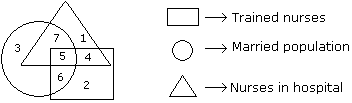Question -

If hospital management requires only married trained nurses for operation theater, which part of diagram should be chosen by him ?

Detailed Solution for Test: Venn Diagrams- 5 - Question 25

because the question is only married trained nurses, so the diagram circle, triangle (represents nurses in hospital) and rectangle where intersect is the married trained nurses to the hospital. So the answer is 5 only.

Test: Venn Diagrams- 5 - Question 26

Directions to Solve

Study the following figure and answer the questions given below.Question -

By which number, married but untrained nurses in the hospital are represented?

Detailed Solution for Test: Venn Diagrams- 5 - Question 26

Correct option is C. 6

Test: Venn Diagrams- 5 - Question 27

Directions to Solve

Study the following figure and answer the questions given below.Question -

By which numbers trained nurses are represented ?

Detailed Solution for Test: Venn Diagrams- 5 - Question 27

Option A = 3, 6 ; Here 3 is 'just only a married population'.
Option B = 7, 5 ; Here 7 is 'married nurses in hospital but not trained'.
Option D = 1, 1 ; Here 1 is 'nurses in hospital but not trained'.

But, Option C = 5, 6 ; Here both 5 & 6 are covered with 'Trained nurses'. Hence the required answers.

Test: Venn Diagrams- 5 - Question 28

Directions to Solve

Study the following figure and answer the questions given below.Question -

What is represented by the number 7 ?

Detailed Solution for Test: Venn Diagrams- 5 - Question 28

Explanation:

• As triangle represents the nurses in hospital.
• And circle represents the married population.
• 7 is in intersecting point of circle and triangle
• So, No 7 repersents mazrried nurses in the hospital.
Test: Venn Diagrams- 5 - Question 29

Directions to Solve

Study the following figure and answer the questions given below.Question -

By which number, the trained unmarried nurses in the hospital are represented ?

Detailed Solution for Test: Venn Diagrams- 5 - Question 29

This is because in the given data states that there are only married, trained nurses in the hospital. But in the question it is asked as whether the nurse is unmarried. Eventually, the answer should be the left out number (the number in the triangle&square). So the answer is 4 only.

Test: Venn Diagrams- 5 - Question 30

Directions to Solve

Study the following figure and answer the questions given below.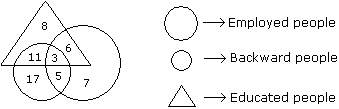Question -

How many educated people are employed ?

Detailed Solution for Test: Venn Diagrams- 5 - Question 30

Number of educated people who are employed = 3 + 6 = 9.

## Logical Reasoning (LR) and Data Interpretation (DI)

98 videos|95 docs|121 tests
Information about Test: Venn Diagrams- 5 Page
In this test you can find the Exam questions for Test: Venn Diagrams- 5 solved & explained in the simplest way possible. Besides giving Questions and answers for Test: Venn Diagrams- 5, EduRev gives you an ample number of Online tests for practice

## Logical Reasoning (LR) and Data Interpretation (DI)

98 videos|95 docs|121 tests

### How to Prepare for CAT

Read our guide to prepare for CAT which is created by Toppers & the best Teachers(Scan QR code)